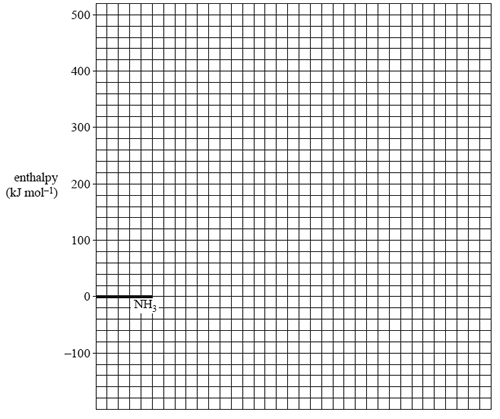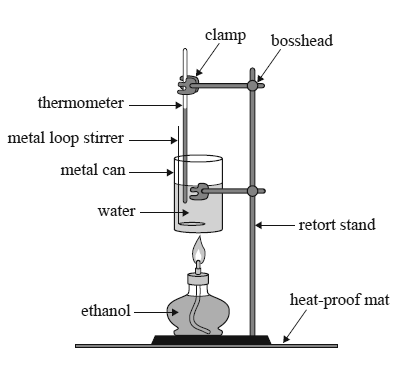Enthalpy (2014 VCE) 1) Large deposits of methane hydrate have been discovered deep under the sediment on the ocean fl oor. It has been suggested that methane hydrate deposits could be commercially mined to provide a clean fuel once the trapped methane is extracted. Methane hydrate has a complex structure. The simplifi ed formula for methane hydrate is CH4.6H2 O. The amount of energy released by the complete combustion of methane extracted from a 1.00 kg sample of methane hydrate at SLC is A. 8.89 × 102 kJ B. 7.17 × 103 kJ C. 4.30 × 104 kJ D. 5.56 × 104 kJ Solution2) The decomposition of ammonia is represented by the following equation. 2NH3(g) => N2(g) + 3H2(g) ΔH = 92.4 kJ mol–1 a. The activation energy for the uncatalysed reaction is 335 kJ mol–1. The activation energy for the reaction when tungsten is used as a catalyst is 163 kJ mol–1. On the grid provided below, draw a labelled energy profile diagram for the uncatalysed and catalysed reactionsSolutionb) When osmium is used as a catalyst, the activation energy is 197 kJ mol–1. Which catalyst – osmium or tungsten – will cause ammonia to decompose at a faster rate? Justify your answer in terms of the chemical principles you have studied this year. Solution2) The enthalpy for the combustion of ethanol is provided in the data book. This combustion of ethanol is represented by the following equation. C2H5OH(l) + 3O2(g) => 2CO2(g) + 3H2O(l) A spirit burner used 1.80 g of ethanol to raise the temperature of 100.0 g of water in a metal can from 25.0 °C to 40.0 °C.Calculate the percentage of heat lost to the environment and to the apparatus. Solutionb) Identify one way to limit heat loss to the environment. Solutionc) Biodiesel may be produced by reacting canola oil with methanol in the presence of a strong base. Since canola oil contains a mixture of triglycerides, the reaction produces glycerol and a mixture of biodiesel molecules. A typical biodiesel molecule derived from canola oil has the chemical formula C15H30O2. Write the semi-structural formula of this molecule, then circle and name the functional group present. Solutiond) The heat content of canola oil can be determined by placing it in the spirit burner in place of ethanol. A typical result is 17 kJ g–1. Suggest why the heat content of fuels such as canola oil and biodiesel are measured in kJ g–1 and not kJ mol–1. Solution